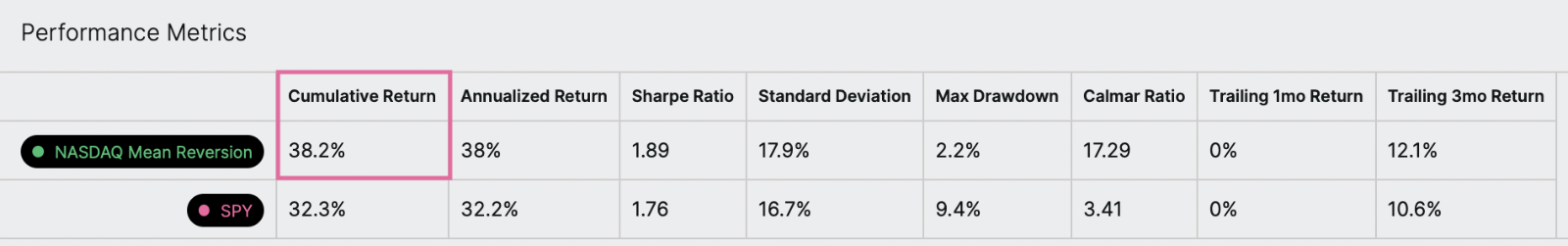# Cumulative return

What is cumulative return?

The cumulative return is the percent change in the value of an investment over a given time period.

An example

Let’s take a look at an example. In this backtest, we see the symphony’s cumulative return is 38.2%. That means that this symphony’s value increased by 38.2% over the length of the backtest period.What is the formula?What is the step-by-step calculation?

Let's go through the calculation of the cumulative return step-by-step for a symphony backtest. Here's how we do it:

• Start with the value of the symphony on the final (i.e., the most recent) trading day of the backtest.
• Subtract the value of the symphony on the first trading day of the backtest.
• Divide by the value of the symphony on the first trading day of the backtest.
• Multiply the resulting value by 100.